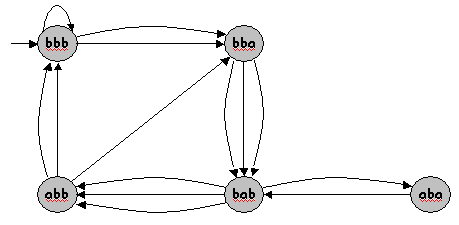# Digest: Text Generation with Markov Models

## Posted on Oct 4 by Anonymous

Imagine if you could write a program to write you an essay, or produce a tweet, or even generate a research paper? Such an activity can be performed with reasonable quality by the use of something known as a Markov Model, with the help of an input text. In this post, I’ll be covering a little about what Markov Models are and how they can be used to generate potentially interesting text.

Disclaimer: I am by no means an expert on this topic, and this post is merely a way of sharing a cool idea I learnt during one of my classes (CS 2020) as a student at NUS.

## Background

A Markov Model is used to model a system that randomly evolves with time, where the future state depends only on the current state, and not on any of the previous states. To clarify what that means, take for example a simple game, such as snakes and ladders. Each position on the board reflects a state, and your next position is completely random (determined by the dice roll). Furthermore, your next position only depends on your current position on the board, and not where you were earlier. Such a process, that evolves randomly with time, is called a stochastic process, and one where the future state depends only on the current state is called a Markov Chain, named after the Russian mathematician Andrey Markov.

A Markov Model is used for such processes, to find the probabilities of being in particular states given an initial state and the probabilities of transitioning from one state to another. So, in the case of snakes of ladders, every state can lead to six new states, each one with a probability of 16.

An example of a Markov Chain from Wikipedia:### Text Generation

Claude Shannon, in his famous paper A Mathematical Theory of Communication (which laid the groundwork of modern information theory), proposed, among other things, using a Markov chain to statistically model the sequences of letters in a piece of English text. The idea is to have every state represented by a k-gram, or a string of length k, and to assign probabilities of transitioning from one k-gram to another, based on the frequency of characters appearing after the k-gram in the input text. Such a model is called a Markov Model of order k.

Here is an example of the idea, for k = 1. Suppose the input text is “a g g c g a g g g a g c g g c a g g g g”  We can construct our Markov Model of order 1 as follows

k-gram(k = 1) Subsequent Character Probability
a g 44
g g 712
g a 212
g c 312
c g 23
c a 13

What this means is that the probability of finding a ‘g’ after an ‘a’ is 1, as in the text, every occurrence of an ‘a’ is followed by a ‘g’. Similarly, the probability of finding a ‘c’ after a g is 23 because that’s the number of times a ‘c’ appears after a ‘g’ in the text, divided by the number of times ‘g’ appears.

In this manner, we can construct the model by finding the probabilities of single characters appearing after each k-gram in the input text. Once that is done, we start from a particular state, and then randomly move to new states based on the probabilities, each movement to a new state represents adding a new character to our generated text.

A Diagrammatic Illustration of Text Generation:You may wonder why such a simple idea like this could give rise to meaningful results. In practice, a lot of it has to do with the value of k. If k is a small value, then the generated text is often one filled with gibberish. On the other hand, if k is too large, the generated text will end up sounding a lot more like the input text. It’s also worth noting that the quality of the text generated depends on the input itself, i.e. you’re more likely to get a better play as your output if the input is a Shakesperean text, than if it is a poltician’s speech.

## Closing Thoughts

Implementing this idea is not hard, and potentially a lot of fun (CS2020 students can testify!) You could also try using words instead of characters, where your k-gram is a string of k words, and you find the probability of a particular word appearing after a given k-gram, rather than a character.

Here are some implementations online of Markov Models:

On a final note, text generation is just one application of Markov Models, they find applications in a large variety of spheres, such as the GeneMark algorithm for gene prediction, the Metropolis algorithm for measuring thermodynamical properties, and Google’s PageRank algorithm.